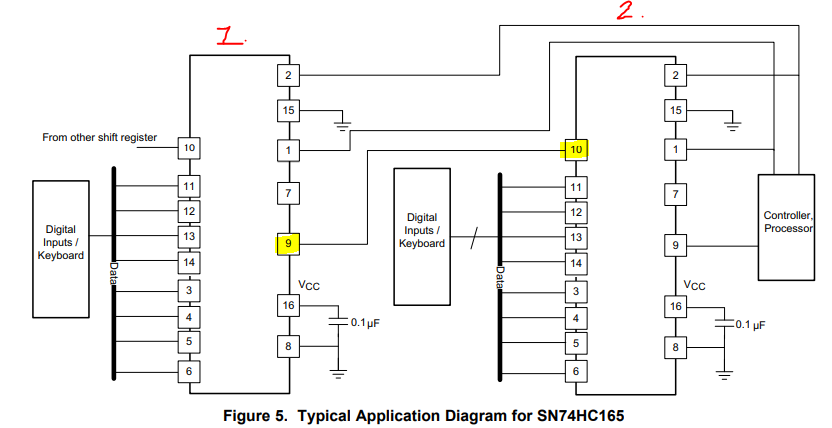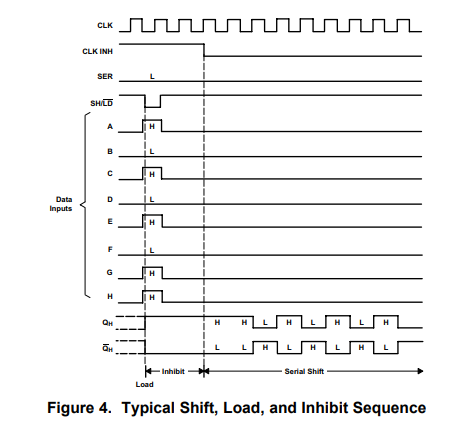If you have a related question, please click the "Ask a related question" button in the top right corner. The newly created question will be automatically linked to this question.

# SN74HC165: SER input function

Part Number: SN74HC165

Hi Team,

My customer is asking what the basic function of SN74HC165 , I think customer can look into d/s figure 4 and Table 1 for reference,

however , there's no example for SER pin's function, could you kindly help for explain how SER pin work?

let says, there's data 11111111(8 bit binary code) shift from device 1 to device 2  , and device 2's parallel input pin(A~H) is carry the bit 10101011 as d/s figure 4 shown, then what would be the series output of the device 2's QH pin when shift function is on(SH/LD#=H , CLK= rising , CLK INH=L)?d/s figure 4.• The combination of the two devices behaves like a 16-bit shift register. The first eight bits are from the inputs of the second device, the following bits are from the first device. In your example, the output is 1101010111111111.

• Hi Clements,

by your mean the first output to the last output bit is from left to right , is that correct?

• I do not know how "left" and "right" would apply to the bits.

As shown in the datasheet, H is first shifted out, A, last.

• Hi Clement ,

I think I get what you meaning , per d/s , H is first shifted out, A, last, so if the output data from left to right is 10101011,

the most left bit is the last bit(from A), the most right bit is the first bit(from H).

and if two device combine together , output data is 1101010111111111 , then the first eight bit been shift out is second device input(10101011) , then following the 8 bits(11111111) from first device output.